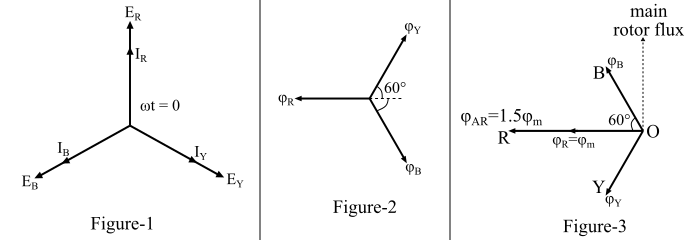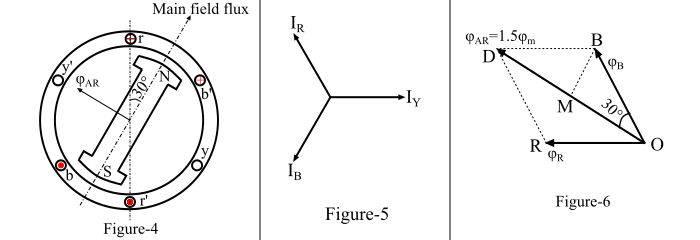# Armature Reaction in Alternator at Unity Power Factor Load

Electronics & ElectricalElectronDigital Electronics

#### Ethical Hacking & Cyber Security Online Training

36 Lectures 5 hours

#### Machine Learning & BIG Data Analytics: Microsoft AZURE

46 Lectures 3.5 hours

#### Advance Big Data Analytics using Hive & Sqoop

Best Seller

50 Lectures 4 hours

Consider the direction of rotation of the rotor is clockwise. The direction of the generated voltage in various conductors can be determined by applying the right-hand rule keeping in view that the direction of rotation of the conductors with respect to the rotor poles is anticlockwise.

Consider the alternator is supplying current at unity power factor. The phase currents 𝐼𝑅 , 𝐼𝑌 and 𝐼𝐵 will be in phase with their respective generated voltage 𝐸𝑅 , 𝐸𝑌 and 𝐸𝐵 as shown in Figure-1. The positive direction of fluxes φ𝑅, φ𝑌 and φB are shown in Figure-2. The projection of a phasor on the vertical axis gives its instantaneous value.Thus, at t = 0, the instantaneous values of currents and fluxes are given by,

$$\mathrm{𝑖_{𝑅} = 𝐼_{𝑚};\:\:φ_{𝑅} = φ_{𝑚}}$$

$$\mathrm{𝑖_{𝑌} = −𝐼_{𝑚}\:cos 60° = −\frac{{𝐼_{𝑚}}}{2};\:\:\:φ_{𝑌} = −\frac{{φ_{𝑚}}}{2}}$$

$$\mathrm{𝑖_{𝐵} = − 𝐼_{𝑚}\:cos 60° = −\frac{{𝐼_{𝑚}}}{2};\:\:\:φ_{B} = −\frac{{φ_{𝑚}}}{2}}$$

Where, $𝐼_{𝑚}$ denotes the maximum value of current and $𝜑_{𝑚}$ denotes the maximum value of flux.

The direction of flux $𝜑_{𝑅}$ is along OR in Figure-3 which is the same direction as in Figure-2. Since the fluxes $𝜑_{Y}$ and $𝜑_{B}$ are negative and hence they act opposite to the directions shown in Figure-2 and these are represented by OY and OB in Figure-3. Thus, the resultant of the fluxes can be found by resolving the fluxes horizontally and vertically.

Resolving the fluxes along the horizontal axis, we obtain,

$$\mathrm{φ_{h} = -{φ_{𝑅}}-{φ_{𝑌}}\:cos\:60° - {φ_{𝐵}} \:cos\:60°}$$

$$\mathrm{\Rightarrow\:φ_{h} = −φ_{𝑚} −(\frac{φ_{𝑚}}{2}) (\frac{1}{2})-(\frac{φ_{𝑚}}{2}) (\frac{1}{2})}$$

$$\mathrm{\Rightarrow\:φ_{ℎ} = −1.5\:φ_{𝑚}}$$

Similarly, resolving the fluxes along the vertical axis, we have,

$$\mathrm{φ_{\nu} = −φ_{𝑌}\:cos\:30° + φ_{𝐵}\:cos \:30°}$$

$$\mathrm{\Rightarrow\:φ_{\nu} = −(\frac{φ_{𝑚}}{2})\:cos\:30°+(\frac{φ_{𝑚}}{2})\:cos\:30°= 0}$$

Therefore, the resultant armature reaction flux is given by,

$$\mathrm{φ_{𝐴𝑅} =\sqrt{φ^{2}_{h}+ φ^{2}_{\nu}}=\sqrt{(−1.5\:φ_{𝑚})^{2} + 0} = 1.5\:φ_{𝑚}}$$

$$\mathrm{\Rightarrow\:φ_{𝐴𝑅} = 1.5\:𝜑_{𝑚}}$$

The direction of this resultant flux is along OR in Figure-3. It can be seen that the resultant armature reaction flux $φ_{𝐴𝑅}$ is constant in magnitude and is equal to 1.5$φ_{𝑚}$. Also, the resultant armature reaction flux lags behind 90° with the main field flux.

Now, consider the case when the rotor has rotated through an angle of 30° in the clockwise direction as shown in Figure-4. The phasor diagram of currents corresponding to this case is shown in Figure-5.Here, at the instant when 𝜔𝑡 = 30°, the instantaneous values of currents and fluxes are given by,

$$\mathrm{𝑖_{𝑅} = 𝐼_{𝑚}\:cos\:30° =\frac{\sqrt{3}}{2}𝐼_{𝑚}; \:\:\:\:φ_{𝑅} =\frac{\sqrt{3}}{2}φ_{𝑚}}$$

$$\mathrm{𝑖_{𝑌} = 0;\:\:\:\:φ_{𝑌} = 0}$$

$$\mathrm{𝑖_{𝐵} = −𝐼_{𝑚}\:cos\:30° = −\frac{\sqrt{3}}{2}𝐼_{𝑚}; \:\:\:\:φ_{B} = −\frac{\sqrt{3}}{2}φ_{𝑚}}$$

Figure-6 shows the space diagram for fluxes at . Thus, the resultant armature reaction flux is given by,

$$\mathrm{φ_{𝐴𝑅} = 𝑂𝐷 = 2𝑂𝑀}$$

$$\mathrm{\Rightarrow\:φ_{𝐴𝑅} = 2 𝑂𝐵\:cos\:30° = 2 \times (\frac{\sqrt{3}}{2}φ_{𝑚})\times (\frac{\sqrt{3}}{2})}$$

$$\mathrm{\Rightarrow\:φ_{𝐴𝑅} = 1.5\:φ_{𝑚}}$$

In this case, the direction of the resultant armature reaction flux $φ_{𝐴𝑅}$ is along OD which makes an angle with horizontal axis in the clockwise direction.

Therefore, it can be seen that the resultant armature flux $φ_{𝐴𝑅}$ produced by the armature currents remains constant in magnitude and is equal to 1.5 $φ_{𝑚}$ and it rotates at synchronous speed. It can also be observed that when the current is in phase with the generated voltage, the resultant armature reaction flux $φ_{𝐴𝑅}$ lags behind the main-field flux by 90° and hence it is called as cross-magnetising flux. If the armature reaction flux is considered to act independently, then this flux will induce EMF in each phase of the alternator which lags the respective phase currents by 90°.

From the above discussion it is clear that when the alternator supplies a load at unity power factor, the effect of the armature reaction is cross-magnetising.